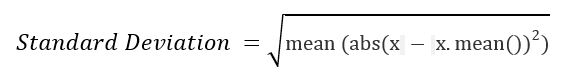# sciPy stats.nanstd() function | Python

• Last Updated : 11 Feb, 2019

`scipy.stats.nanstd(array, axis=0)` function calculates the standard deviation by ignoring the Nan (not a number) values of the array elements along the specified axis of the array.

It’s formula –Parameters :
array : Input array or object having the elements, including Nan values, to calculate the standard deviation.
axis : Axis along which the standard deviation is to be computed. By default axis = 0

Returns : Standard deviation of the array elements (ignoring the Nan values) based on the set parameters.

Code #1:

 `# standard deviation ``import` `scipy``import` `numpy as np`` ` `arr1 ``=` `[``1``, ``3``, np.nan, ``27``] ``  ` `print``(``"standard deviation using nanstd :"``, scipy.nanstd(arr1))`` ` `print``(``"standard deviation without handling nan value :"``, scipy.std(arr1)) `

Output :

```standard deviation using nanstd : 11.813363431112899
standard deviation without handling nan value : nan```

Code #2: With multi-dimensional data

 `# standard deviation ``from` `scipy ``import` `std``from` `scipy ``import` `nanstd``import` `numpy as np`` ` `arr1 ``=` `[[``1``, ``3``, ``27``], ``        ``[``3``, np.nan, ``6``], ``        ``[np.nan, ``6``, ``3``], ``        ``[``3``, ``6``, np.nan]] ``  ` `print``(``"standard deviation is :"``, std(arr1)) ``print``(``"standard deviation handling nan :"``, nanstd(arr1)) `` ` `# using axis = 0``print``(``"\nstandard deviation is with default axis = 0 : \n"``, ``      ``std(arr1, axis ``=` `0``))``print``(``"\nstandard deviation handling nan with default axis = 0 : \n"``, ``      ``nanstd(arr1, axis ``=` `0``))`` ` `# using axis = 1``print``(``"\nstandard deviation is with default axis = 1 : \n"``, ``      ``std(arr1, axis ``=` `1``))  ``print``(``"\nstandard deviation handling nan with default axis = 1 : \n"``, ``      ``nanstd(arr1, axis ``=` `1``))  `

Output :

```standard deviation is : nan
standard deviation handling nan : 7.455216087651669

standard deviation is with default axis =0 :
[nan nan nan]

standard deviation handling nan with default axis =0 :
[ 0.94280904  1.41421356 10.67707825]

standard deviation is with default axis =1 :
[11.81336343         nan         nan         nan]

standard deviation handling nan with default axis =1 :
[11.81336343  1.5         1.5         1.5       ]```

My Personal Notes arrow_drop_up